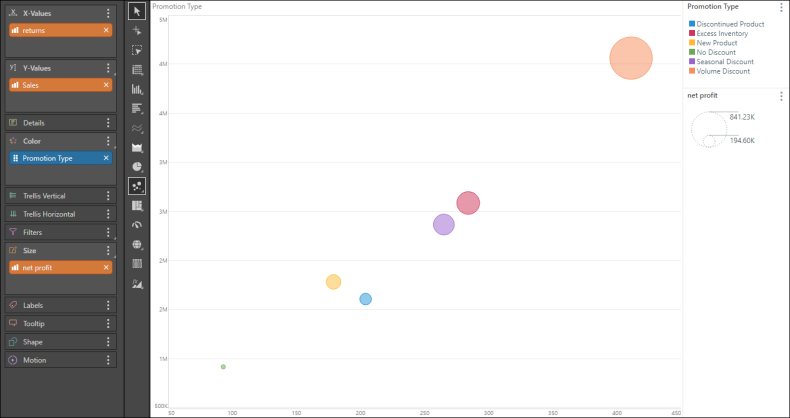# Bubble Chart

Bubble charts use the first three columns of a query to plot the x, y and z axes (and then every subsequent set of two columns). The rows of the query, which are typically used as the basis for the categories of a chart, are displayed as data points in the plot.

The z-axis in this case is represented by the SIZE of the bubbles - add the measure that should influence the size of the data points to the size drop zone.

The bubble chart required a measure in the X-Values drop zone, a second measure in the Y-Values zone, and a third measure in the Size zone. It also requires a member hierarchy in the Color zone.

NOTE: A bubble plot chart cannot be viewed with fewer than three columns of data.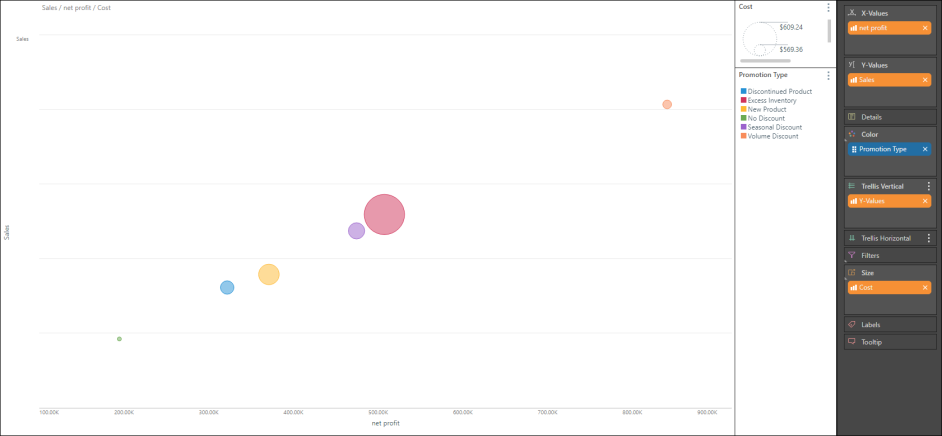## Build a Bubble Chart

##### Step 1

Add the required member hierarchy to the Color drop zone.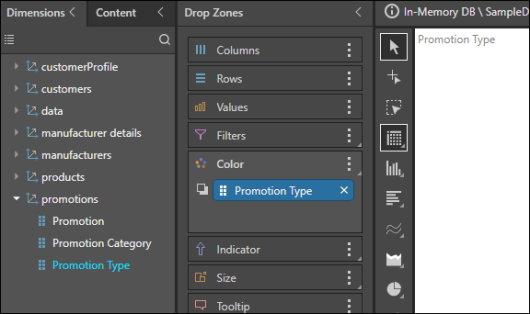##### Step 2

Select Bubble Chart from the Scatter Chart fly-out menu.##### Step 3

To the X-Values drop zone, add the measure that should be on the x-axis.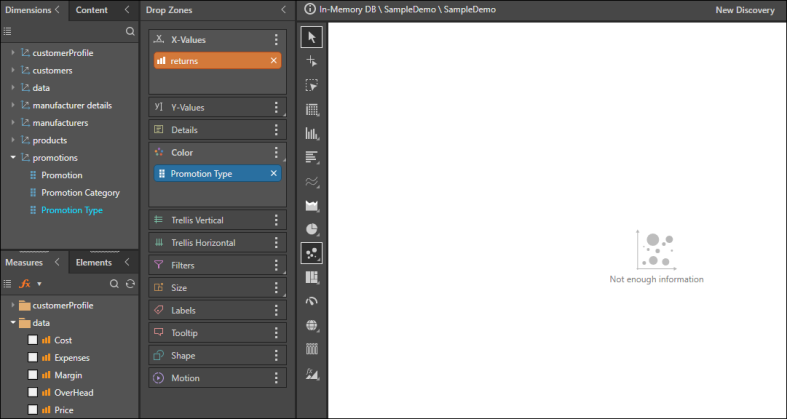##### Step 4

To the Y-Values drop zone, add the measure that should be on the y-axis.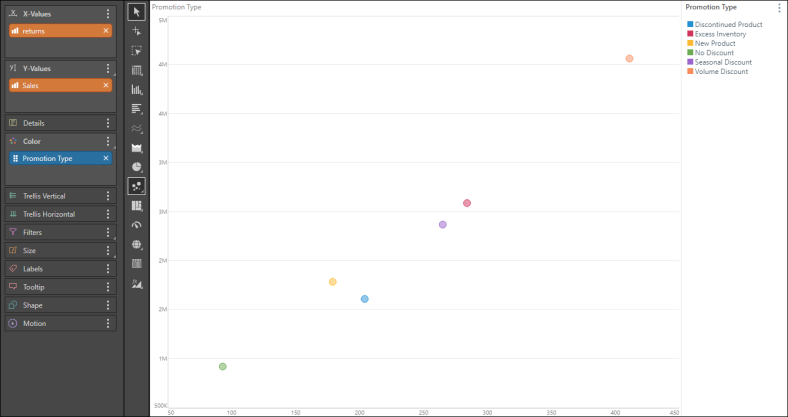##### Step 5

To the Size zone, add the measure that should drive the size of the bubble chart's data points.